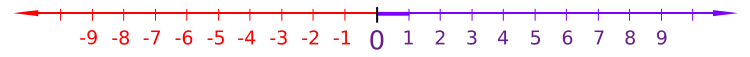# Discrete groupThe integers with their usual topology are a discrete subgroup of the real numbers.

In mathematics, a discrete subgroup of a topological group G is a subgroup H such that there is an open cover of H in which every open subset contains exactly one element of H; in other words, the subspace topology of H in G is the discrete topology. For example, the integers, Z, form a discrete subgroup of the reals, R (with the standard metric topology), but the rational numbers, Q, do not. A discrete group is a topological group G equipped with the discrete topology.

Any group can be given the discrete topology. Since every map from a discrete space is continuous, the topological homomorphisms between discrete groups are exactly the group homomorphisms between the underlying groups. Hence, there is an isomorphism between the category of groups and the category of discrete groups. Discrete groups can therefore be identified with their underlying (non-topological) groups.

There are some occasions when a topological group or Lie group is usefully endowed with the discrete topology, 'against nature'. This happens for example in the theory of the Bohr compactification, and in group cohomology theory of Lie groups.

A discrete isometry group is an isometry group such that for every point of the metric space the set of images of the point under the isometries is a discrete set. A discrete symmetry group is a symmetry group that is a discrete isometry group.

## Properties

Since topological groups are homogeneous, one need only look at a single point to determine if the topological group is discrete. In particular, a topological group is discrete if and only if the singleton containing the identity is an open set.

A discrete group is the same thing as a zero-dimensional Lie group (uncountable discrete groups are not second-countable so authors who require Lie groups to satisfy this axiom do not regard these groups as Lie groups). The identity component of a discrete group is just the trivial subgroup while the group of components is isomorphic to the group itself.

Since the only Hausdorff topology on a finite set is the discrete one, a finite Hausdorff topological group must necessarily be discrete. It follows that every finite subgroup of a Hausdorff group is discrete.

A discrete subgroup H of G is cocompact if there is a compact subset K of G such that HK = G.

Discrete normal subgroups play an important role in the theory of covering groups and locally isomorphic groups. A discrete normal subgroup of a connected group G necessarily lies in the center of G and is therefore abelian.

Other properties:

• every discrete group is totally disconnected
• every subgroup of a discrete group is discrete.
• every quotient of a discrete group is discrete.
• the product of a finite number of discrete groups is discrete.
• a discrete group is compact if and only if it is finite.
• every discrete group is locally compact.
• every discrete subgroup of a Hausdorff group is closed.
• every discrete subgroup of a compact Hausdorff group is finite.

## Examples

• Frieze groups and wallpaper groups are discrete subgroups of the isometry group of the Euclidean plane. Wallpaper groups are cocompact, but Frieze groups are not.
• A crystallographic group usually means a cocompact, discrete subgroup of the isometries of some Euclidean space. Sometimes, however, a crystallographic group can be a cocompact discrete subgroup of a nilpotent or solvable Lie group.
• Every triangle group T is a discrete subgroup of the isometry group of the sphere (when T is finite), the Euclidean plane (when T has a Z + Z subgroup of finite index), or the hyperbolic plane.
• Fuchsian groups are, by definition, discrete subgroups of the isometry group of the hyperbolic plane.
• A Fuchsian group that preserves orientation and acts on the upper half-plane model of the hyperbolic plane is a discrete subgroup of the Lie group PSL(2,R), the group of orientation preserving isometries of the upper half-plane model of the hyperbolic plane.
• A Fuchsian group is sometimes considered as a special case of a Kleinian group, by embedding the hyperbolic plane isometrically into three-dimensional hyperbolic space and extending the group action on the plane to the whole space.
• The modular group PSL(2,Z) is thought of as a discrete subgroup of PSL(2,R). The modular group is a lattice in PSL(2,R), but it is not cocompact.
• Kleinian groups are, by definition, discrete subgroups of the isometry group of hyperbolic 3-space. These include quasi-Fuchsian groups.
• A Kleinian group that preserves orientation and acts on the upper half space model of hyperbolic 3-space is a discrete subgroup of the Lie group PSL(2,C), the group of orientation preserving isometries of the upper half-space model of hyperbolic 3-space.
• A lattice in a Lie group is a discrete subgroup such that the Haar measure of the quotient space is finite.